# VOLTAGE DIVISION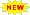Click or Tap the Example circuits below to invoke TINACloud and select the Interactive DC mode to Analyze them Online.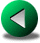WYE to DELTA and DELTA to WYE CONVERSIONCURRENT DIVISION

A series connected circuit is often referred to as a voltage divider circuit. The source voltage equals the total of all voltage drops across the series connected resistors. The voltage dropped across each resistor is proportional to the resistance value of that resistor. Larger resistors experience larger drops, while smaller resistors experience smaller drops. The voltage divider formula allows you to calculate the voltage drop across any resistor without having to first solve for the current. The voltage divider formula is: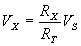where VX = voltage dropped across selected resistor

RX = selected resistor’s value

RT = total series circuit resistance

VS = source or applied voltage

A simple example to start:

Example 1

Find the voltage drop across each resistor, given that V=150 V, R = 1 Kohm.

Click/tap the circuit above to analyze on-line or click this link to Save under Windows

The first solution requires that we find the series current. First, calculate the circuit’s total resistance: Rtot = R1 + R2 = 1k+2k = 3 kohm.

Next, find the circuit current: I = V/Rtot = 150/3 = 50 mA.

Finally, find the voltage across R1: V1= I R1 = 50 V;

and the voltage across R2: V2 = I R2 = 100 V.

The second, more direct solution uses the voltage divider formula: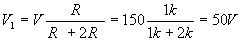and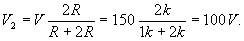{Solution by TINA's Interpreter!}

I:=V/(R+2*R);

VR:=I*R;

V2R:=I*2*R;

VR=

V2R=

{or using the voltage divider formula:}

VR:=V*R/(R+2*R);

V2R:=V*2*R/(R+2*R);

VR=

V2R=

Another example:

Example 2

Click/tap the circuit above to analyze on-line or click this link to Save under Windows

Find the voltage drop on each resistors.

Use the voltage divider formula: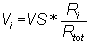{Solution by TINA's Interpreter!}

{Use the voltage divider formula: Vi= Vs*Ri/Rtot}

V1:=VS*R1/(R1+R2+R3+R4);

V2:=VS*R2/(R1+R2+R3+R4);

V3:=VS*R3/(R1+R2+R3+R4);

V4:=VS*R4/(R1+R2+R3+R4);

V1=[500m]

V2=

V3=[1.5]

V4=

Example 3

Find the voltages measured by the instruments.

Click/tap the circuit above to analyze on-line or click this link to Save under Windows

This example shows that the branch connected in parallel with the source does not affect the use of the voltage division formula.

{Solution by TINA's Interpreter}

V1:=V*R3/(R3+R4);

V1=

V2:=V*R4/(R3+R4);

V2=

The following example is a bit more complicated:

Example 4

Click/tap the circuit above to analyze on-line or click this link to Save under Windows

Find the voltage drop across R2 if the voltage source is 140 V and the resistances are as given in the schematic.

{Solution by TINA's Interpreter!}

V4:=Vs*(Replus(R4,(R2+R3)))/(R1+Replus((R2+R3),R4));

V:=V4*R2/(R2+R3)

{or}

Sys I,I2,I1,V

I*R4=I2*(R2+R3)

I1=I+I2

V=I2*R2

Vs=R1*I1+I*R4

end;

V=

The voltage division formula is used twice, first to find the voltage across R4, and second to find the voltage across R2.

Example 5

Click/tap the circuit above to analyze on-line or click this link to Save under Windows

Find the voltage between the nodes A and B.

Use the voltage division formula three times: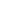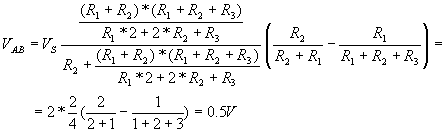The method here is to first find the voltage between the ground node and the node (2) where R2, R3, and R1 are joined. This is done using the voltage divider formula to find the portion of Vs appearing between these two nodes. Then the voltage divider formula is used twice to find Va and Vb. Finally, Vb is subtracted from Va.

{Solution by TINA' Interpreter!}

R12:=Replus((R1+R2),(R1+R2+R3));

V12:=Vs*R12/(R2+R12);

Vab:=V12*(R2/(R1+R2)-R1/(R1+R2+R3));

Vab=[500m]WYE to DELTA and DELTA to WYE CONVERSIONCURRENT DIVISION